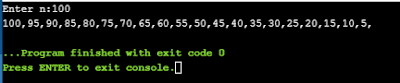## Pages

### While loop - Python practice programs

While loop - Python practice programs:

Copy and try on your own.
1) Find first n numbers divisible by 5 using while loop

code:
'''first n numbers divisible by 5'''
n=int(input("Enter n:"))
while(n>0):
if n%5 == 0:
print(n, end=',')

n-=1First n number divisible by 5 using while loop
Output:Output of first n number divisible by 5